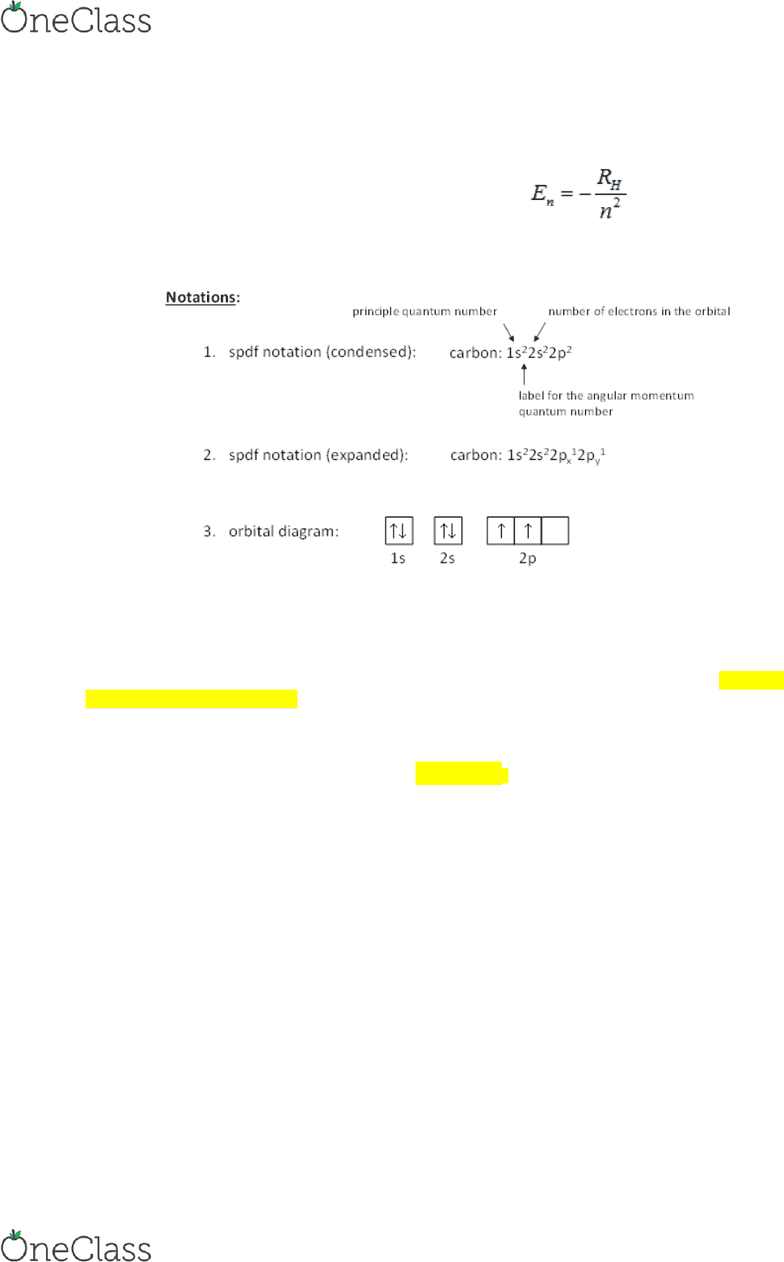Study Guides (400,000)
CA (160,000)
Queen's (5,000)
CHEM (80)
Midterm

# CHEM 112 Study Guide - Midterm Guide: Strontium, Bromine, Gallium

Department
Chemistry
Course Code
CHEM 112
Professor
John Carran
Study Guide
Midterm

This preview shows half of the first page. to view the full 3 pages of the document.Unit 2 Chemistry
Energy of an orbital in the hydrogen atom is:
where RH = 2.179 10-18 J is the Rydberg energy.
The Photoelectric Effect
Electrons are emitted from the surface of a metal when it's exposed to light. This is called the photoelectric effect.
Each metal has a certain threshold frequency of light, below which nothing happens. Right at this threshold
frequency, an electron is emitted. Above this frequency, the electron is emitted and the extra energy is transferred to
the electron.
The equation for this phenomenon is
KE=0
where KE is the kinetic energy of the emitted electron, h=6.63×10−34Js is Planck's constant, ν is the frequency
of the light, and ν0 is the threshold frequency of the metal.
Also, since E=, the equation can also be written as
KE=EE0
where E is the energy of the light and E0 is the threshold energy of the metal.
PRACTICE QUESTIONS
1. Which of the following statements is (are) correct for an electron with n = 6 and ml = 2?
a. The electron may be in a d orbital.
find more resources at oneclass.com
find more resources at oneclass.com